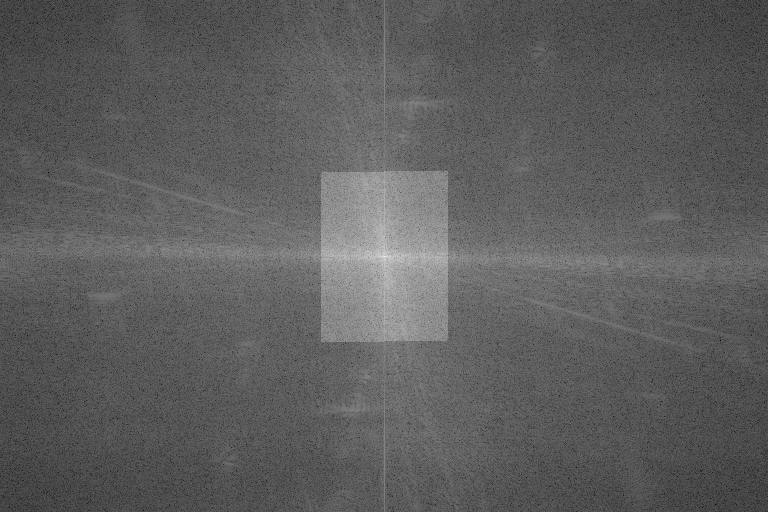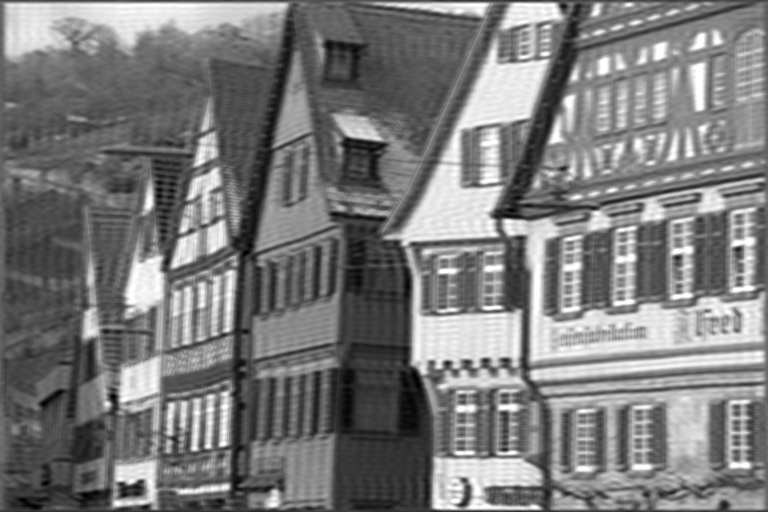Inverse FFT

# Overview

Inverse FFT implements the inverse Fourier Transform for 2D images, supporting real- and complex-valued outputs. Given a 2D spectrum (frequency domain), it returns the image representation on the spatial domain. It is the exact inverse of FFT algorithm.

Input as a magnitude spectrum Output in spatial domain# Implementation

The inverse Fast Fourier Transform follows closely forward FFT's implementation, except for the sign of the exponent, which is positive here.

$I[m,n] = \frac{1}{MN} \sum^{M-1}_{u=0} \sum^{N-1}_{v=0} I'[u,v] e^{+2\pi i (\frac{um}{M}+\frac{vn}{N})}$

Where:

• $$I'$$ is the input image in frequency domain
• $$I$$ is its spatial domain representation
• $$M\times N$$ is input's dimensions

The normalization factor $$\frac{1}{MN}$$ is applied by default to make IFFT an exact inverse of FFT. As this incurs in a performance hit and normalization might not be needed, user can pass the flag VPI_DENORMALIZED_OUTPUT to vpiSubmitIFFT to indicate that output must be left denormalized.

As with direct FFT, depending on $$N$$, different techniques are employed for best performance:

• CPU backend
• Fast paths when $$N$$ can be factored into $$2^a \times 3^b \times 5^c$$
• CUDA backend
• Fast paths when $$N$$ can be factored into $$2^a \times 3^b \times 5^c \times 7^d$$

In general the smaller the prime factor is, the better the performance, i.e., powers of two are fastest.

IFFT supports the following transform types:

• complex-to-complex, C2C
• complex-to-real, C2R.

## Data Layout

Data layout depends strictly on the transform type. In case of general C2C transform, both input and output data shall be of type VPI_IMAGE_FORMAT_2F32 and have the same size. In C2R mode, each input image row $$(X_1,X_2,\dots,X_{\lfloor\frac{N}{2}\rfloor+1})$$ of type VPI_IMAGE_FORMAT_2F32 containing only non-redundant values results in row $$(x_1,x_2,\dots,x_N)$$ with type VPI_IMAGE_FORMAT_F32 representing the whole image. In both cases, input and output image heights are the same.

Note
There can be memory allocation in first call to vpiSubmitIFFT and every time the input or output row stride changes with respect to previous call.

# C API functions

For list of limitations, constraints and backends that implements the algorithm, consult reference documentation of the following functions:

Function Description
vpiCreateIFFT Creates payload for inverse Fast Fourier Transform algorithm.
vpiSubmitIFFT Runs the inverse Fast Fourier Transform on single image.

# Usage

Language:
1. Import VPI module
import vpi
2. Run the C2R IFFT on a VPI image input with spectrum data in vpi.Format._2F32 format. The resulting VPI image has vpi.Format.F32 format. Operation is executed by the CUDA backend.
with vpi.Backend.CUDA:
output = input.irfft()
1. Initialization phase
1. Include the header that defines the inverse FFT functions.
#include <vpi/algo/FFT.h>
Declares functions that implement the Fast Fourier Transform algorithm and its inverse.
2. Define the input spectrum image. Since we're performing a C2R IFFT, the input width must be $$\lfloor \frac{w}{2} \rfloor+1$$, where $$w$$ is the output image width. Input and output heights must match. Image format must be VPI_IMAGE_FORMAT_2F32.
VPIImage input = /*...*/;
struct VPIImageImpl * VPIImage
A handle to an image.
Definition: Types.h:256
3. Create the output image. Again, because of C2F IFFT, the output type must be VPI_IMAGE_FORMAT_F32. We're passing 0 as flags to make the function return a normalized output.
VPIImage output;
#define VPI_IMAGE_FORMAT_F32
Single plane with one 32-bit floating point channel.
Definition: ImageFormat.h:130
VPIStatus vpiImageCreate(int32_t width, int32_t height, VPIImageFormat fmt, uint64_t flags, VPIImage *img)
Create an empty image instance with the specified flags.
4. Create the IFFT payload on the CUDA backend. Input is complex and output is real. Problem size refers to output size.
#define VPI_IMAGE_FORMAT_2F32
Single plane with two interleaved 32-bit floating point channels.
Definition: ImageFormat.h:136
VPIStatus vpiCreateIFFT(uint64_t backends, int32_t outputWidth, int32_t outputHeight, const VPIImageFormat inFormat, const VPIImageFormat outFormat, VPIPayload *payload)
Creates payload for inverse Fast Fourier Transform algorithm.
A handle to an algorithm payload.
Definition: Types.h:268
@ VPI_BACKEND_CUDA
CUDA backend.
Definition: Types.h:93
5. Create the stream where the algorithm will be submitted for execution.
VPIStream stream;
vpiStreamCreate(0, &stream);
struct VPIStreamImpl * VPIStream
A handle to a stream.
Definition: Types.h:250
VPIStatus vpiStreamCreate(uint64_t flags, VPIStream *stream)
Create a stream instance.
2. Processing phase
1. Submit the IFFT algorithm to the stream, passing the input spectrum and the output buffer. It'll be executed on the CUDA backend, since the payload is created there.
vpiSubmitIFFT(stream, VPI_BACKEND_CUDA, ifft, input, output, 0);
VPIStatus vpiSubmitIFFT(VPIStream stream, uint64_t backend, VPIPayload payload, VPIImage input, VPIImage output, uint64_t flags)
Runs the inverse Fast Fourier Transform on single image.
2. Wait until the processing is done.
vpiStreamSync(stream);
VPIStatus vpiStreamSync(VPIStream stream)
Blocks the calling thread until all submitted commands in this stream queue are done (queue is empty)...
3. Now display output using your preferred method.
3. Cleanup phase
1. Free resources held by the stream, the payload and the input and output images.
vpiImageDestroy(output);
void vpiImageDestroy(VPIImage img)
Destroy an image instance.